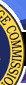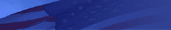Home | Previous Page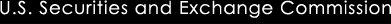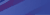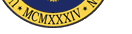# Rate of Return

The annual rate of return is the percentage change in the value of an investment.

For example: If you assume you earn a 10% annual rate of return, then you are assuming that the value of your investment will increase by 10% every year. So, if you invest \$1,000 for 1 year, then your investment would be worth \$1,100 at the end of the one year period, before subtracting expenses.

http://www.sec.gov/investor/tools/mfcc/rate-of-return-help.htm